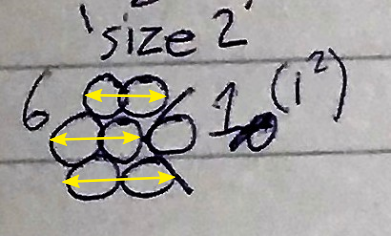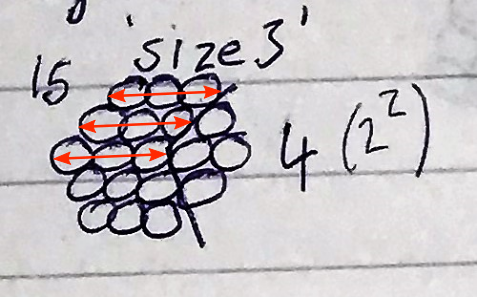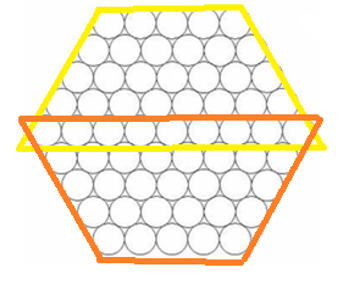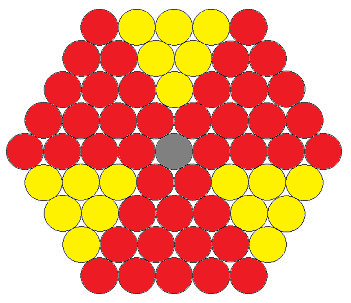#### You may also like### Just Rolling Round

P is a point on the circumference of a circle radius r which rolls, without slipping, inside a circle of radius 2r. What is the locus of P?### Coke Machine

The coke machine in college takes 50 pence pieces. It also takes a certain foreign coin of traditional design...### Just Opposite

A and C are the opposite vertices of a square ABCD, and have coordinates (a,b) and (c,d), respectively. What are the coordinates of the vertices B and D? What is the area of the square?

# Steel Cables

##### Age 14 to 16Challenge Level

Rohan from Stephen Perse Foundation in the UK used this geometrical method:

I started by taking 'size 2' and 'size 3' hexagons.

If you split the spapes into two like below, you can easily find the $n$th term for each.If you take the part on the right, you can see it is a square number. It seems to be the square of the number one less than the 'size', therefore $(n-1)^2$.

For the part on the left, there are a certain number of rows, with 'side' on each row. Therefore, it is a multiple of $n.$ For 'size' $2,$ it is $3\times n.$ For 'size' $3,$ it is $5\times n.$ $3$ is $2\times2-1$ and $5$ is $3\times2-1.$ Therefore it is $2n-1$ multiplied by $n,$ or $n(2n-1)$ or $2n^2-n.$

Combining the two together you get $2n^2-n+(n-1)^2.$

Sanika from India used a similar but different method:

I divided the hexagon into 2 trapeziums like the picture shows:I then assumed that 1 circle represented 1 unit and calculated the area of both the trapeziums using the formula $2\times\left(h\frac{N + b}2\right).$ I then proceeded to subtract [$b$ from the result] as it was added twice. So the final equation was $h(N + b)-b.$ So $N$ would be the size of the cable, $b$ would be $2N-1$ and $h$ would again be the size of the cable as it represents the height.

So in a size $10$ cable, $N$ would be $10,$ $b$ would be $19$ and $h$ would be $10.$ Inserting these values into our formula, we get $10(29)-19= 271.$

Brady from Canada sent us a similar solution:We can break a size $n$ hexagon into $3$ big triangles (of side length $n$) and 3 small triangles (of side length $n-2$). Since the $3$ big triangles meet in the middle, when we add up the number of strands in each we have to subtract $2$ because we have overcounted the middle strand by $2$:

$\frac{3n(n+1)}{2}$ + $\frac{3(n-2)(n-1)}{2}- 2 = 3n^2 - 3n + 1$

Calvin from Ethiopia and Patrick from Otterbourne used an algebraic approach:

At first I noticed a pattern: the number of strands per cable was going up by multiples of 6 every time. Then I plotted the data for sizes 1-10:

 Size 1 2 3 4 5 6 7 8 9 10 Strands 1 7 19 37 61 91 127 169 217 271

and graphed them. The result was a parabola, so I found its equation and that was the answer. The answer is s = 3n2 - 3n + 1, where s is the number of strands required and n is the size of the cable.

Patrick described a method for finding Calvin's formula:

The sequence starts off: 1, 7, 19, 37, 61, ...
If we take the difference between successive terms, we get the sequence 6, 12, 18, 24, ...
If we take successive differences again, we get 6, 6, 6, ...

This is constant, so we must have a quadratic equation s = an$^2$ + bn + c.
In fact, half of 6 is 3, so we know that a = 3. Therefore

s = 3n$^2$ + bn + c

In order to find b and c, we can substitute values in:

(n = 1, s = 1) 1 = 3 + b + c

(n = 2, s = 7) 7 = 12 + 2b + c

and we can solve these simultaneous equations to get b = -3 and c = 1.

Sanika also used an algebraic aproach to check the result from the trapezium method:

To verify, I developed a slightly similar method, but this was without using the formula for the area of a trapezium. I again divided the hexagon into $2$ trapeziums and noticed that there would be $N$ rows in each trapezium wherein, consecutive rows will have one more strand than the previous one. Therefore, the total number of strands would be $(N, N+1, N+2, N+3 .......N + (N-1)).$ Then I proceeded to simplify this using the formula used to find the sum of consecutive numbers.

Sum of consecutive numbers = $\frac x 2 (\text{first number + last number})$

So the value of $x$ here would be the value on $N$ as it stands for the number of terms we are summing up. Substituting $N$ into this formula will give us $\frac{N}{2}(3N-1).$

Since we have $2$ trapeziums, we will multiply this by $2$ and subtract the $(2N-1)$ from the result as it is common between the two trapeziums.

This gives us a final formula of $N(3N-1) – (2N-1).$ Substituting for $N,$ I got $10(29)-19=271$ which was the same result I got earlier.

Therefore the number of strands needed can be given by the formula $N(3N-1) – (2N-1),$ where $N$ is the size of the cable.

Well spotted! This formula was discovered by a mathematician called Gauss, who probably used something like Sanika's trapeziums to figure it out.